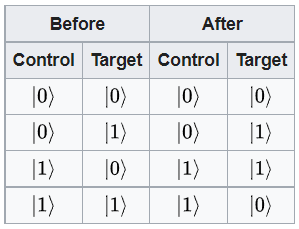• Members 4 posts

Is it possible to say that every controlled operation,could entangled qubits
And if not ,what special about cnot

• Members 12 posts

The short answer is no, not every controlled operation can entangle (or unentangle) the qubits.

To understand why, let us briefly discuss:
1. What "entangled" means
2. What controlled operations do

1. What "entangled" means

Entanglement describes the quantum state of two or more qubits. Take the simple 2-qubit quantum state as examples. When two qubits are unentangled, the quantum state of the two qubits are "independent", in the sense that measurement on qubit 1 will not impact the measurement result of qubit 2. So that the total quantum state can be expressed as a tensor product of two single-qubit state:
$$\ket{\psi_{tot}} = \ket{\psi_1} \otimes \ket{\psi_2}$$

When 2 qubits are entangled, the measurement of one qubit can determine the result of the other, even though each of them have some probability of being 0 and 1 before being measured. The total quantum state can no longer be described by the tensor product of two single-qubit state. The following are examples of entangled state, also called Bell states, which are the maximally entangled state for 2 qubits.
$$\ket{\phi_0} = \frac{1}{\sqrt{2}}(\ket{00} + \ket{11}), \ket{\phi_1} = \frac{1}{\sqrt{2}}(\ket{00} - \ket{11}), \ket{\phi_2} = \frac{1}{\sqrt{2}}(\ket{01} + \ket{10}), \ket{\phi_3} = \frac{1}{\sqrt{2}}(\ket{01} - \ket{10}),$$

2. What controlled operations do

Control operations are not directly related to entangling the state. By definition, controlled operations act on target qubits, based on the value of control qubits. Take CNOT for example, the control qubit can decide whether a NOT gate will operate on the target qubit - if the control gate is 0, does nothing; if the control gate is 1, operate NOT gate on target qubit. The possible results are summarized below(image from Wikipedia)

What's special in quantum computation is that, when input an entangled state, the CNOT operation can change each of the superposition state in a way depending on the target qubit, for example:
$$\ket{\phi_0} = \frac{1}{\sqrt{2}}(\ket{00} + \ket{11}) \rightarrow \frac{1}{\sqrt{2}}(\ket{00} + \ket{10}) = \frac{1}{\sqrt{2}}(\ket{0} + \ket{1}) \otimes \ket{0}.$$
CNOT happens to change the entangled 2-qubit states into unentangled states. But this is not a general property for all controlled operations.

image.png

PNG, 9.7 KB, uploaded by SchrodingersMouse on Nov. 8, 2021.